# NMR Restraints Grid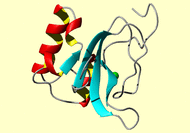Result table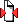(Save to zip file containing files for each block)

 image mrblock_id pdb_id bmrb_id cing stage position program type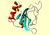496386 2l30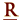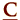17157 cing 1-original 2 AMBER dihedral angle

```# 1679 atoms read from pdb file 01.pdb.
# 9 TYR PHI:  (8 LEU C)-(9 TYR N)-(9 TYR CA)-(9 TYR C) -168.3 -106.4
&rst     iat =   118,   120,   122,   139,
r1 = -169.3, r2 = -168.3, r3 = -106.4, r4 = -105.4,
rk2 =   100.0, rk3 =   100.0,    &end

# 9 TYR PSI:  (9 TYR N)-(9 TYR CA)-(9 TYR C)-(10 ARG N) 132.6 168.4
&rst     iat =   120,   122,   139,   141,
r1 = 131.6, r2 = 132.6, r3 = 168.4, r4 = 169.4,
&end

# 10 ARG PHI:  (9 TYR C)-(10 ARG N)-(10 ARG CA)-(10 ARG C) -161.5 -82.6
&rst     iat =   139,   141,   143,   163,
r1 = -162.5, r2 = -161.5, r3 = -82.6, r4 = -81.6,
&end

# 10 ARG PSI:  (10 ARG N)-(10 ARG CA)-(10 ARG C)-(11 VAL N) 118.1 142.1
&rst     iat =   141,   143,   163,   165,
r1 = 117.1, r2 = 118.1, r3 = 142.1, r4 = 143.1,
&end

# 11 VAL PHI:  (10 ARG C)-(11 VAL N)-(11 VAL CA)-(11 VAL C) -169.6 -95.1
&rst     iat =   163,   165,   167,   179,
r1 = -170.6, r2 = -169.6, r3 = -95.1, r4 = -94.1,
&end

# 11 VAL PSI:  (11 VAL N)-(11 VAL CA)-(11 VAL C)-(12 GLU N) 130.6 175.7
&rst     iat =   165,   167,   179,   181,
r1 = 129.6, r2 = 130.6, r3 = 175.7, r4 = 176.7,
&end

# 15 LYS PHI:  (14 ALA C)-(15 LYS N)-(15 LYS CA)-(15 LYS C) -113.3 -58.7
&rst     iat =   225,   227,   229,   247,
r1 = -114.3, r2 = -113.3, r3 = -58.7, r4 = -57.7,
&end

# 15 LYS PSI:  (15 LYS N)-(15 LYS CA)-(15 LYS C)-(16 SER N) -46.6 -25.9
&rst     iat =   227,   229,   247,   249,
r1 = -47.6, r2 = -46.6, r3 = -25.9, r4 = -24.9,
&end

# 16 SER PHI:  (15 LYS C)-(16 SER N)-(16 SER CA)-(16 SER C) -168.7 -76.6
&rst     iat =   247,   249,   251,   258,
r1 = -169.7, r2 = -168.7, r3 = -76.6, r4 = -75.6,
&end

# 16 SER PSI:  (16 SER N)-(16 SER CA)-(16 SER C)-(17 GLY N) 132.9 165.9
&rst     iat =   249,   251,   258,   260,
r1 = 131.9, r2 = 132.9, r3 = 165.9, r4 = 166.9,
&end

# 27 SER PHI:  (26 GLU C)-(27 SER N)-(27 SER CA)-(27 SER C) -128.9 -58.3
&rst     iat =   400,   402,   404,   411,
r1 = -129.9, r2 = -128.9, r3 = -58.3, r4 = -57.3,
&end

# 27 SER PSI:  (27 SER N)-(27 SER CA)-(27 SER C)-(28 ILE N) 101.8 144.2
&rst     iat =   402,   404,   411,   413,
r1 = 100.8, r2 = 101.8, r3 = 144.2, r4 = 145.2,
&end

# 28 ILE PHI:  (27 SER C)-(28 ILE N)-(28 ILE CA)-(28 ILE C) -127.1 -49.1
&rst     iat =   411,   413,   415,   430,
r1 = -128.1, r2 = -127.1, r3 = -49.1, r4 = -48.1,
&end

# 28 ILE PSI:  (28 ILE N)-(28 ILE CA)-(28 ILE C)-(29 PRO N) 107.5 138.8
&rst     iat =   413,   415,   430,   432,
r1 = 106.5, r2 = 107.5, r3 = 138.8, r4 = 139.8,
&end

# 29 PRO PSI:  (29 PRO N)-(29 PRO CA)-(29 PRO C)-(30 LYS N) 128.4 157.2
&rst     iat =   432,   442,   444,   446,
r1 = 127.4, r2 = 128.4, r3 = 157.2, r4 = 158.2,
&end

# 33 LEU PHI:  (32 SER C)-(33 LEU N)-(33 LEU CA)-(33 LEU C) -114.6 -60.0
&rst     iat =   489,   491,   493,   508,
r1 = -115.6, r2 = -114.6, r3 = -60.0, r4 = -59.0,
&end

# 33 LEU PSI:  (33 LEU N)-(33 LEU CA)-(33 LEU C)-(34 ARG N) 118.8 139.8
&rst     iat =   491,   493,   508,   510,
r1 = 117.8, r2 = 118.8, r3 = 139.8, r4 = 140.8,
&end

# 34 ARG PHI:  (33 LEU C)-(34 ARG N)-(34 ARG CA)-(34 ARG C) -147.6 -87.0
&rst     iat =   508,   510,   512,   532,
r1 = -148.6, r2 = -147.6, r3 = -87.0, r4 = -86.0,
&end

# 34 ARG PSI:  (34 ARG N)-(34 ARG CA)-(34 ARG C)-(35 MET N) 128.9 161.2
&rst     iat =   510,   512,   532,   534,
r1 = 127.9, r2 = 128.9, r3 = 161.2, r4 = 162.2,
&end

# 35 MET PHI:  (34 ARG C)-(35 MET N)-(35 MET CA)-(35 MET C) -146.2 -106.2
&rst     iat =   532,   534,   536,   549,
r1 = -147.2, r2 = -146.2, r3 = -106.2, r4 = -105.2,
&end

# 35 MET PSI:  (35 MET N)-(35 MET CA)-(35 MET C)-(36 ALA N) 127.2 148.3
&rst     iat =   534,   536,   549,   551,
r1 = 126.2, r2 = 127.2, r3 = 148.3, r4 = 149.3,
&end

# 36 ALA PHI:  (35 MET C)-(36 ALA N)-(36 ALA CA)-(36 ALA C) -161.4 -90.7
&rst     iat =   549,   551,   553,   559,
r1 = -162.4, r2 = -161.4, r3 = -90.7, r4 = -89.7,
&end

# 36 ALA PSI:  (36 ALA N)-(36 ALA CA)-(36 ALA C)-(37 ILE N) 126.4 166.3
&rst     iat =   551,   553,   559,   561,
r1 = 125.4, r2 = 126.4, r3 = 166.3, r4 = 167.3,
&end

# 37 ILE PHI:  (36 ALA C)-(37 ILE N)-(37 ILE CA)-(37 ILE C) -132.7 -90.3
&rst     iat =   559,   561,   563,   578,
r1 = -133.7, r2 = -132.7, r3 = -90.3, r4 = -89.3,
&end

# 37 ILE PSI:  (37 ILE N)-(37 ILE CA)-(37 ILE C)-(38 MET N) 128.5 148.5
&rst     iat =   561,   563,   578,   580,
r1 = 127.5, r2 = 128.5, r3 = 148.5, r4 = 149.5,
&end

# 38 MET PHI:  (37 ILE C)-(38 MET N)-(38 MET CA)-(38 MET C) -133.9 -80.9
&rst     iat =   578,   580,   582,   595,
r1 = -134.9, r2 = -133.9, r3 = -80.9, r4 = -79.9,
&end

# 38 MET PSI:  (38 MET N)-(38 MET CA)-(38 MET C)-(39 VAL N) 103.1 131.2
&rst     iat =   580,   582,   595,   597,
r1 = 102.1, r2 = 103.1, r3 = 131.2, r4 = 132.2,
&end

# 39 VAL PHI:  (38 MET C)-(39 VAL N)-(39 VAL CA)-(39 VAL C) -158.5 -86.1
&rst     iat =   595,   597,   599,   611,
r1 = -159.5, r2 = -158.5, r3 = -86.1, r4 = -85.1,
&end

# 39 VAL PSI:  (39 VAL N)-(39 VAL CA)-(39 VAL C)-(40 GLN N) 125.9 157.7
&rst     iat =   597,   599,   611,   613,
r1 = 124.9, r2 = 125.9, r3 = 157.7, r4 = 158.7,
&end

# 40 GLN PHI:  (39 VAL C)-(40 GLN N)-(40 GLN CA)-(40 GLN C) -146.6 -44.7
&rst     iat =   611,   613,   615,   628,
r1 = -147.6, r2 = -146.6, r3 = -44.7, r4 = -43.7,
&end

# 40 GLN PSI:  (40 GLN N)-(40 GLN CA)-(40 GLN C)-(41 SER N) 102.6 144.5
&rst     iat =   613,   615,   628,   630,
r1 = 101.6, r2 = 102.6, r3 = 144.5, r4 = 145.5,
&end

# 41 SER PHI:  (40 GLN C)-(41 SER N)-(41 SER CA)-(41 SER C) -117.5 -42.6
&rst     iat =   628,   630,   632,   639,
r1 = -118.5, r2 = -117.5, r3 = -42.6, r4 = -41.6,
&end

# 41 SER PSI:  (41 SER N)-(41 SER CA)-(41 SER C)-(42 PRO N) 105.1 156.7
&rst     iat =   630,   632,   639,   641,
r1 = 104.1, r2 = 105.1, r3 = 156.7, r4 = 157.7,
&end

# 47 LYS PHI:  (46 GLY C)-(47 LYS N)-(47 LYS CA)-(47 LYS C) -161.9 -88.4
&rst     iat =   709,   711,   713,   731,
r1 = -162.9, r2 = -161.9, r3 = -88.4, r4 = -87.4,
&end

# 47 LYS PSI:  (47 LYS N)-(47 LYS CA)-(47 LYS C)-(48 VAL N) 135.2 155.6
&rst     iat =   711,   713,   731,   733,
r1 = 134.2, r2 = 135.2, r3 = 155.6, r4 = 156.6,
&end

# 48 VAL PHI:  (47 LYS C)-(48 VAL N)-(48 VAL CA)-(48 VAL C) -161.6 -105.1
&rst     iat =   731,   733,   735,   747,
r1 = -162.6, r2 = -161.6, r3 = -105.1, r4 = -104.1,
&end

# 48 VAL PSI:  (48 VAL N)-(48 VAL CA)-(48 VAL C)-(49 PRO N) 106.4 161.1
&rst     iat =   733,   735,   747,   749,
r1 = 105.4, r2 = 106.4, r3 = 161.1, r4 = 162.1,
&end

# 49 PRO PSI:  (49 PRO N)-(49 PRO CA)-(49 PRO C)-(50 HIE N) 120.7 146.9
&rst     iat =   749,   759,   761,   763,
r1 = 119.7, r2 = 120.7, r3 = 146.9, r4 = 147.9,
&end

# 50 HIS PHI:  (49 PRO C)-(50 HIE N)-(50 HIE CA)-(50 HIE C) -140.0 -71.3
&rst     iat =   761,   763,   765,   778,
r1 = -141.0, r2 = -140.0, r3 = -71.3, r4 = -70.3,
&end

# 50 HIS PSI:  (50 HIE N)-(50 HIE CA)-(50 HIE C)-(51 TRP N)  96.0 158.1
&rst     iat =   763,   765,   778,   780,
r1 =  95.0, r2 =  96.0, r3 = 158.1, r4 = 159.1,
&end

# 51 TRP PHI:  (50 HIE C)-(51 TRP N)-(51 TRP CA)-(51 TRP C) -157.9 -78.9
&rst     iat =   778,   780,   782,   802,
r1 = -158.9, r2 = -157.9, r3 = -78.9, r4 = -77.9,
&end

# 51 TRP PSI:  (51 TRP N)-(51 TRP CA)-(51 TRP C)-(52 TYR N) 127.8 172.2
&rst     iat =   780,   782,   802,   804,
r1 = 126.8, r2 = 127.8, r3 = 172.2, r4 = 173.2,
&end

# 52 TYR PHI:  (51 TRP C)-(52 TYR N)-(52 TYR CA)-(52 TYR C) -177.2 -63.7
&rst     iat =   802,   804,   806,   823,
r1 = -178.2, r2 = -177.2, r3 = -63.7, r4 = -62.7,
&end

# 52 TYR PSI:  (52 TYR N)-(52 TYR CA)-(52 TYR C)-(53 HIE N) 146.2 166.2
&rst     iat =   804,   806,   823,   825,
r1 = 145.2, r2 = 146.2, r3 = 166.2, r4 = 167.2,
&end

# 54 PHE PHI:  (53 HIE C)-(54 PHE N)-(54 PHE CA)-(54 PHE C) -83.9 -43.9
&rst     iat =   840,   842,   844,   860,
r1 = -84.9, r2 = -83.9, r3 = -43.9, r4 = -42.9,
&end

# 54 PHE PSI:  (54 PHE N)-(54 PHE CA)-(54 PHE C)-(55 SER N) -53.6 -33.6
&rst     iat =   842,   844,   860,   862,
r1 = -54.6, r2 = -53.6, r3 = -33.6, r4 = -32.6,
&end

# 55 SER PHI:  (54 PHE C)-(55 SER N)-(55 SER CA)-(55 SER C) -84.3 -44.3
&rst     iat =   860,   862,   864,   871,
r1 = -85.3, r2 = -84.3, r3 = -44.3, r4 = -43.3,
&end

# 55 SER PSI:  (55 SER N)-(55 SER CA)-(55 SER C)-(56 CYM N) -48.2 -28.2
&rst     iat =   862,   864,   871,   873,
r1 = -49.2, r2 = -48.2, r3 = -28.2, r4 = -27.2,
&end

# 56 CYS PHI:  (55 SER C)-(56 CYM N)-(56 CYM CA)-(56 CYM C) -81.2 -41.2
&rst     iat =   871,   873,   875,   881,
r1 = -82.2, r2 = -81.2, r3 = -41.2, r4 = -40.2,
&end

# 56 CYS PSI:  (56 CYM N)-(56 CYM CA)-(56 CYM C)-(57 PHE N) -55.9 -35.9
&rst     iat =   873,   875,   881,   883,
r1 = -56.9, r2 = -55.9, r3 = -35.9, r4 = -34.9,
&end

# 57 PHE PHI:  (56 CYM C)-(57 PHE N)-(57 PHE CA)-(57 PHE C) -83.8 -43.8
&rst     iat =   881,   883,   885,   901,
r1 = -84.8, r2 = -83.8, r3 = -43.8, r4 = -42.8,
&end

# 57 PHE PSI:  (57 PHE N)-(57 PHE CA)-(57 PHE C)-(58 TRP N) -51.2 -31.2
&rst     iat =   883,   885,   901,   903,
r1 = -52.2, r2 = -51.2, r3 = -31.2, r4 = -30.2,
&end

# 58 TRP PHI:  (57 PHE C)-(58 TRP N)-(58 TRP CA)-(58 TRP C) -107.0 -46.4
&rst     iat =   901,   903,   905,   925,
r1 = -108.0, r2 = -107.0, r3 = -46.4, r4 = -45.4,
&end

# 58 TRP PSI:  (58 TRP N)-(58 TRP CA)-(58 TRP C)-(59 LYS N) -29.7   1.1
&rst     iat =   903,   905,   925,   927,
r1 = -30.7, r2 = -29.7, r3 =   1.1, r4 =   2.1,
&end

# 59 LYS PHI:  (58 TRP C)-(59 LYS N)-(59 LYS CA)-(59 LYS C) -110.8 -32.3
&rst     iat =   925,   927,   929,   947,
r1 = -111.8, r2 = -110.8, r3 = -32.3, r4 = -31.3,
&end

# 59 LYS PSI:  (59 LYS N)-(59 LYS CA)-(59 LYS C)-(60 VAL N) -41.6 -17.9
&rst     iat =   927,   929,   947,   949,
r1 = -42.6, r2 = -41.6, r3 = -17.9, r4 = -16.9,
&end

# 60 VAL PHI:  (59 LYS C)-(60 VAL N)-(60 VAL CA)-(60 VAL C) -123.7 -49.4
&rst     iat =   947,   949,   951,   963,
r1 = -124.7, r2 = -123.7, r3 = -49.4, r4 = -48.4,
&end

# 60 VAL PSI:  (60 VAL N)-(60 VAL CA)-(60 VAL C)-(61 GLY N) -46.9  -3.8
&rst     iat =   949,   951,   963,   965,
r1 = -47.9, r2 = -46.9, r3 =  -3.8, r4 =  -2.8,
&end

# 62 HIS PHI:  (61 GLY C)-(62 HIE N)-(62 HIE CA)-(62 HIE C) -129.8 -60.3
&rst     iat =   970,   972,   974,   987,
r1 = -130.8, r2 = -129.8, r3 = -60.3, r4 = -59.3,
&end

# 62 HIS PSI:  (62 HIE N)-(62 HIE CA)-(62 HIE C)-(63 SER N) 128.5 151.7
&rst     iat =   972,   974,   987,   989,
r1 = 127.5, r2 = 128.5, r3 = 151.7, r4 = 152.7,
&end

# 63 SER PHI:  (62 HIE C)-(63 SER N)-(63 SER CA)-(63 SER C) -143.5 -50.4
&rst     iat =   987,   989,   991,   998,
r1 = -144.5, r2 = -143.5, r3 = -50.4, r4 = -49.4,
&end

# 63 SER PSI:  (63 SER N)-(63 SER CA)-(63 SER C)-(64 ILE N) 117.3 154.0
&rst     iat =   989,   991,   998,  1000,
r1 = 116.3, r2 = 117.3, r3 = 154.0, r4 = 155.0,
&end

# 64 ILE PHI:  (63 SER C)-(64 ILE N)-(64 ILE CA)-(64 ILE C) -154.2 -72.1
&rst     iat =   998,  1000,  1002,  1017,
r1 = -155.2, r2 = -154.2, r3 = -72.1, r4 = -71.1,
&end

# 64 ILE PSI:  (64 ILE N)-(64 ILE CA)-(64 ILE C)-(65 ARG N) 119.3 139.3
&rst     iat =  1000,  1002,  1017,  1019,
r1 = 118.3, r2 = 119.3, r3 = 139.3, r4 = 140.3,
&end

# 67 PRO PSI:  (67 PRO N)-(67 PRO CA)-(67 PRO C)-(68 ASP N) -40.7 -16.7
&rst     iat =  1060,  1070,  1072,  1074,
r1 = -41.7, r2 = -40.7, r3 = -16.7, r4 = -15.7,
&end

# 68 ASP PHI:  (67 PRO C)-(68 ASP N)-(68 ASP CA)-(68 ASP C) -84.8 -44.8
&rst     iat =  1072,  1074,  1076,  1084,
r1 = -85.8, r2 = -84.8, r3 = -44.8, r4 = -43.8,
&end

# 68 ASP PSI:  (68 ASP N)-(68 ASP CA)-(68 ASP C)-(69 VAL N) -32.6 -10.0
&rst     iat =  1074,  1076,  1084,  1086,
r1 = -33.6, r2 = -32.6, r3 = -10.0, r4 =  -9.0,
&end

# 69 VAL PHI:  (68 ASP C)-(69 VAL N)-(69 VAL CA)-(69 VAL C) -141.7 -37.6
&rst     iat =  1084,  1086,  1088,  1100,
r1 = -142.7, r2 = -141.7, r3 = -37.6, r4 = -36.6,
&end

# 69 VAL PSI:  (69 VAL N)-(69 VAL CA)-(69 VAL C)-(70 GLU N) -46.4 -19.5
&rst     iat =  1086,  1088,  1100,  1102,
r1 = -47.4, r2 = -46.4, r3 = -19.5, r4 = -18.5,
&end

# 71 VAL PHI:  (70 GLU C)-(71 VAL N)-(71 VAL CA)-(71 VAL C) -129.5 -48.8
&rst     iat =  1115,  1117,  1119,  1131,
r1 = -130.5, r2 = -129.5, r3 = -48.8, r4 = -47.8,
&end

# 71 VAL PSI:  (71 VAL N)-(71 VAL CA)-(71 VAL C)-(72 ASP N)  73.6 113.3
&rst     iat =  1117,  1119,  1131,  1133,
r1 =  72.6, r2 =  73.6, r3 = 113.3, r4 = 114.3,
&end

# 72 ASP PHI:  (71 VAL C)-(72 ASP N)-(72 ASP CA)-(72 ASP C) -141.7 -15.6
&rst     iat =  1131,  1133,  1135,  1143,
r1 = -142.7, r2 = -141.7, r3 = -15.6, r4 = -14.6,
&end

# 72 ASP PSI:  (72 ASP N)-(72 ASP CA)-(72 ASP C)-(73 GLY N) 119.9 143.6
&rst     iat =  1133,  1135,  1143,  1145,
r1 = 118.9, r2 = 119.9, r3 = 143.6, r4 = 144.6,
&end

# 74 PHE PHI:  (73 GLY C)-(74 PHE N)-(74 PHE CA)-(74 PHE C) -75.6 -35.6
&rst     iat =  1150,  1152,  1154,  1170,
r1 = -76.6, r2 = -75.6, r3 = -35.6, r4 = -34.6,
&end

# 74 PHE PSI:  (74 PHE N)-(74 PHE CA)-(74 PHE C)-(75 SER N) -58.8 -26.7
&rst     iat =  1152,  1154,  1170,  1172,
r1 = -59.8, r2 = -58.8, r3 = -26.7, r4 = -25.7,
&end

# 75 SER PHI:  (74 PHE C)-(75 SER N)-(75 SER CA)-(75 SER C) -87.9 -47.9
&rst     iat =  1170,  1172,  1174,  1181,
r1 = -88.9, r2 = -87.9, r3 = -47.9, r4 = -46.9,
&end

# 75 SER PSI:  (75 SER N)-(75 SER CA)-(75 SER C)-(76 GLU N) -30.0  -6.3
&rst     iat =  1172,  1174,  1181,  1183,
r1 = -31.0, r2 = -30.0, r3 =  -6.3, r4 =  -5.3,
&end

# 76 GLU PHI:  (75 SER C)-(76 GLU N)-(76 GLU CA)-(76 GLU C) -135.4 -56.2
&rst     iat =  1181,  1183,  1185,  1196,
r1 = -136.4, r2 = -135.4, r3 = -56.2, r4 = -55.2,
&end

# 76 GLU PSI:  (76 GLU N)-(76 GLU CA)-(76 GLU C)-(77 LEU N) -30.3  19.5
&rst     iat =  1183,  1185,  1196,  1198,
r1 = -31.3, r2 = -30.3, r3 =  19.5, r4 =  20.5,
&end

# 78 ARG PHI:  (77 LEU C)-(78 ARG N)-(78 ARG CA)-(78 ARG C) -143.0 -21.9
&rst     iat =  1215,  1217,  1219,  1239,
r1 = -144.0, r2 = -143.0, r3 = -21.9, r4 = -20.9,
&end

# 78 ARG PSI:  (78 ARG N)-(78 ARG CA)-(78 ARG C)-(79 TRP N) 141.6 166.9
&rst     iat =  1217,  1219,  1239,  1241,
r1 = 140.6, r2 = 141.6, r3 = 166.9, r4 = 167.9,
&end

# 79 TRP PHI:  (78 ARG C)-(79 TRP N)-(79 TRP CA)-(79 TRP C) -77.6 -37.6
&rst     iat =  1239,  1241,  1243,  1263,
r1 = -78.6, r2 = -77.6, r3 = -37.6, r4 = -36.6,
&end

# 79 TRP PSI:  (79 TRP N)-(79 TRP CA)-(79 TRP C)-(80 ASP N) -53.6 -33.6
&rst     iat =  1241,  1243,  1263,  1265,
r1 = -54.6, r2 = -53.6, r3 = -33.6, r4 = -32.6,
&end

# 80 ASP PHI:  (79 TRP C)-(80 ASP N)-(80 ASP CA)-(80 ASP C) -81.3 -41.3
&rst     iat =  1263,  1265,  1267,  1275,
r1 = -82.3, r2 = -81.3, r3 = -41.3, r4 = -40.3,
&end

# 80 ASP PSI:  (80 ASP N)-(80 ASP CA)-(80 ASP C)-(81 ASP N) -50.8 -30.8
&rst     iat =  1265,  1267,  1275,  1277,
r1 = -51.8, r2 = -50.8, r3 = -30.8, r4 = -29.8,
&end

# 81 ASP PHI:  (80 ASP C)-(81 ASP N)-(81 ASP CA)-(81 ASP C) -85.7 -45.7
&rst     iat =  1275,  1277,  1279,  1287,
r1 = -86.7, r2 = -85.7, r3 = -45.7, r4 = -44.7,
&end

# 81 ASP PSI:  (81 ASP N)-(81 ASP CA)-(81 ASP C)-(82 GLN N) -50.6 -30.6
&rst     iat =  1277,  1279,  1287,  1289,
r1 = -51.6, r2 = -50.6, r3 = -30.6, r4 = -29.6,
&end

# 82 GLN PHI:  (81 ASP C)-(82 GLN N)-(82 GLN CA)-(82 GLN C) -82.3 -42.3
&rst     iat =  1287,  1289,  1291,  1304,
r1 = -83.3, r2 = -82.3, r3 = -42.3, r4 = -41.3,
&end

# 82 GLN PSI:  (82 GLN N)-(82 GLN CA)-(82 GLN C)-(83 GLN N) -51.8 -31.8
&rst     iat =  1289,  1291,  1304,  1306,
r1 = -52.8, r2 = -51.8, r3 = -31.8, r4 = -30.8,
&end

# 83 GLN PHI:  (82 GLN C)-(83 GLN N)-(83 GLN CA)-(83 GLN C) -84.8 -44.8
&rst     iat =  1304,  1306,  1308,  1321,
r1 = -85.8, r2 = -84.8, r3 = -44.8, r4 = -43.8,
&end

# 83 GLN PSI:  (83 GLN N)-(83 GLN CA)-(83 GLN C)-(84 LYS N) -47.5 -27.5
&rst     iat =  1306,  1308,  1321,  1323,
r1 = -48.5, r2 = -47.5, r3 = -27.5, r4 = -26.5,
&end

# 84 LYS PHI:  (83 GLN C)-(84 LYS N)-(84 LYS CA)-(84 LYS C) -84.2 -44.2
&rst     iat =  1321,  1323,  1325,  1343,
r1 = -85.2, r2 = -84.2, r3 = -44.2, r4 = -43.2,
&end

# 84 LYS PSI:  (84 LYS N)-(84 LYS CA)-(84 LYS C)-(85 VAL N) -54.3 -34.3
&rst     iat =  1323,  1325,  1343,  1345,
r1 = -55.3, r2 = -54.3, r3 = -34.3, r4 = -33.3,
&end

# 85 VAL PHI:  (84 LYS C)-(85 VAL N)-(85 VAL CA)-(85 VAL C) -84.3 -44.3
&rst     iat =  1343,  1345,  1347,  1359,
r1 = -85.3, r2 = -84.3, r3 = -44.3, r4 = -43.3,
&end

# 85 VAL PSI:  (85 VAL N)-(85 VAL CA)-(85 VAL C)-(86 LYS N) -52.5 -32.5
&rst     iat =  1345,  1347,  1359,  1361,
r1 = -53.5, r2 = -52.5, r3 = -32.5, r4 = -31.5,
&end

# 86 LYS PHI:  (85 VAL C)-(86 LYS N)-(86 LYS CA)-(86 LYS C) -81.2 -41.1
&rst     iat =  1359,  1361,  1363,  1381,
r1 = -82.2, r2 = -81.2, r3 = -41.1, r4 = -40.1,
&end

# 86 LYS PSI:  (86 LYS N)-(86 LYS CA)-(86 LYS C)-(87 LYS N) -48.9 -28.9
&rst     iat =  1361,  1363,  1381,  1383,
r1 = -49.9, r2 = -48.9, r3 = -28.9, r4 = -27.9,
&end

# 87 LYS PHI:  (86 LYS C)-(87 LYS N)-(87 LYS CA)-(87 LYS C) -82.0 -42.0
&rst     iat =  1381,  1383,  1385,  1403,
r1 = -83.0, r2 = -82.0, r3 = -42.0, r4 = -41.0,
&end

# 87 LYS PSI:  (87 LYS N)-(87 LYS CA)-(87 LYS C)-(88 THR N) -50.5 -30.5
&rst     iat =  1383,  1385,  1403,  1405,
r1 = -51.5, r2 = -50.5, r3 = -30.5, r4 = -29.5,
&end

# 88 THR PHI:  (87 LYS C)-(88 THR N)-(88 THR CA)-(88 THR C) -84.2 -44.2
&rst     iat =  1403,  1405,  1407,  1417,
r1 = -85.2, r2 = -84.2, r3 = -44.2, r4 = -43.2,
&end

# 88 THR PSI:  (88 THR N)-(88 THR CA)-(88 THR C)-(89 ALA N) -51.8 -31.8
&rst     iat =  1405,  1407,  1417,  1419,
r1 = -52.8, r2 = -51.8, r3 = -31.8, r4 = -30.8,
&end

# 89 ALA PHI:  (88 THR C)-(89 ALA N)-(89 ALA CA)-(89 ALA C) -86.3 -46.3
&rst     iat =  1417,  1419,  1421,  1427,
r1 = -87.3, r2 = -86.3, r3 = -46.3, r4 = -45.3,
&end

# 89 ALA PSI:  (89 ALA N)-(89 ALA CA)-(89 ALA C)-(90 GLU N) -39.2 -11.7
&rst     iat =  1419,  1421,  1427,  1429,
r1 = -40.2, r2 = -39.2, r3 = -11.7, r4 = -10.7,
&end

# 90 GLU PHI:  (89 ALA C)-(90 GLU N)-(90 GLU CA)-(90 GLU C) -86.9 -46.9
&rst     iat =  1427,  1429,  1431,  1442,
r1 = -87.9, r2 = -86.9, r3 = -46.9, r4 = -45.9,
&end

# 90 GLU PSI:  (90 GLU N)-(90 GLU CA)-(90 GLU C)-(91 ALA N) -45.4 -15.3
&rst     iat =  1429,  1431,  1442,  1444,
r1 = -46.4, r2 = -45.4, r3 = -15.3, r4 = -14.3,
&end

```

Please acknowledge these references in publications where the data from this site have been utilized.

Contact the webmaster for help, if required. Tuesday, July 5, 2022 12:35:23 PM GMT (wattos1)